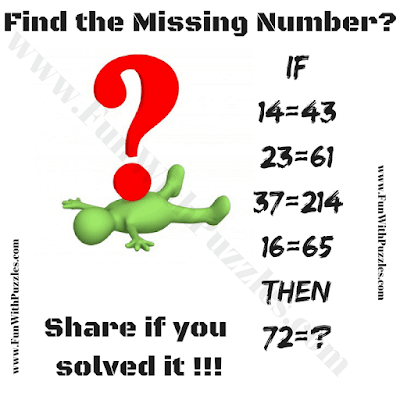Are you ready to put your logical reasoning and math skills to the test? Our Logic Math Problem is designed to challenge your intellect and puzzle-solving abilities. In this intriguing puzzle, you'll encounter a series of equations that may not be correct mathematically, but they are indeed correct logically. Your mission: decipher the logical patterns and solve the riddle.Decode the Logical Equations and Solve the Riddle

The puzzle statement reads: "If 14=43, 23=61, 37=214, 16=65, Then 72=?". At first glance, these equations may appear perplexing, but there's a logical reasoning behind them waiting to be uncovered. To solve this puzzle, you'll need to think critically and analytically.

Logical reasoning is a valuable skill that extends beyond mathematics. It involves making connections, recognizing patterns, and drawing conclusions based on evidence. This puzzle offers an engaging way to exercise your logical thinking and problem-solving abilities.

Now, let's dive into the logic math challenge. Within this series of equations, each number is transformed logically into another. Can you unravel the logic behind it and solve the final equation, "72=?" Time is of the essence, so put on your thinking cap and see how quickly you can crack this Math Puzzle!

The answer to this Logic Math Problem can be viewed by clicking on the button. Please do give your best try before looking at the answer.

#### 1 comment:

Unknown said...

145 is the answer you multiply the numbers and then subtrtact